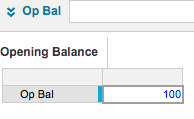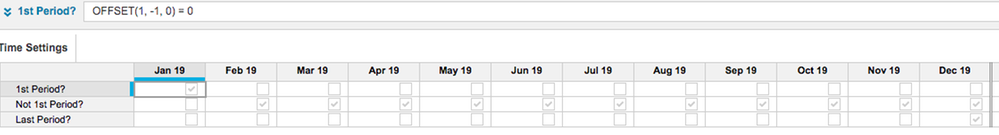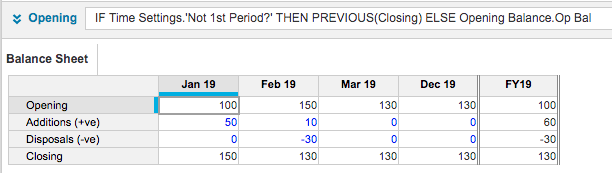# Best practice for 'Corkscrew accounts'Hi,

What is the best practice way to do a classic brought forward / movements / carried forward calculation? Using OFFSET in the brought forward formula to capture the previous period? Or is there a better way?

TIA

Tagged:

•@rob_marshall

Does PREVIOUS perform better than OFFSET as well? My understanding was that PREVIOUS is only better than CUMULATE...

•In this example PREVIOUS would be the preferred formula over OFFSET as it is a simple progression from one period to the next.

OFFSET would be preferred if you were looking at retrieving a value more than 1 period away.

I would suspect that PREVIOUS would also be a more efficient formula for the outcomes you are looking for.
•Previous is the way that you described the problem to it makes the formula easier to understand

Here's a pro-tip to avoid having an extra line to hold the Opening Balance

1. Create a module that doesn't have time to contain the Opening Balances if you can2. In a Time Settings module, calculate the first period and the other periods (and the last one if you need to)3. Now create the Opening / Closing calculation.  Note it is more efficient to have the most common condition firstI've also set the time summary options for Opening and Closing to Opening Balance and Closing Balance respectively

I hope this helps

David

•I did not consider setting up the 1st opening balance in my answer.

@DavidSmiths solution uses a combination of OFFSET and PREVIOUS.

OFFSET(1,-1,0) = 0 has a very specific purpose here. It is determining whether that line item has any periods preceding it -

( the 1 refers to period 1 which is the start of the offset and the -1 the period immediately prior to period 1 which is the destination. It is attempting to retrieve the value of the cell 1 period in the past from the current period. If this is not possible a zero will be returned. As this is impossible for the 1st period then zero is the answer which as the whole formula equals zero will return a true result - a tick in the box ).

This then sets up the conditions possible to populate the first opening balance with PREVIOUS being used to pull these balance forward to the next period.

•That was my first thought but the very first opening balance needs to be established which requires someway of determining where the time period range starts.

OFFSET(1,-1,0)=0 determines the existence of a time period immediately prior to the opening balance. if the time period is the the first in the range then a zero will be returned and the formula will resolve to a TRUE boolean result if it is not then the formula will resolve to FALSE.

An alternative to OFFSET is PREVIOUS('1')=0

'1' is an additional line item

•It's a good point, but the challenge we've see with Brought forward is that the time period doesn't actually exist!  It's hard to map data in and out of it, so we have tended to avoid using it. But in some cases it works, so thanks for mentioning it as an option

As with a lot things in Anaplan there are many ways to achieve the same result - The challenge, and what we've articulated with PLANS is the Best practices and when to use one technique over another

David